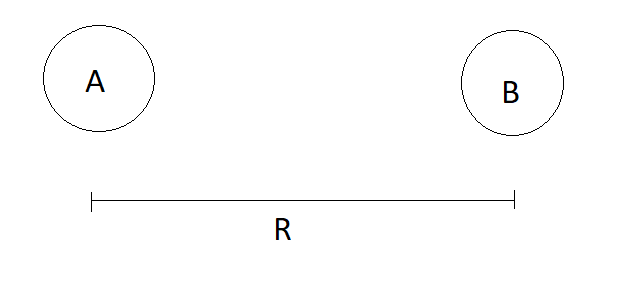# It's net gravitational is 0

After posting this problem I got an interesting result which can be stated as follows:

If two objects are separated by a distance of $R$ and if ratio of the mass of the mass of the second object is $n$ times the mass of the first object then, if any object regardless of it's mass is placed at a distance of $\boxed{\frac{R}{\sqrt{n}+1}}$ from the first object the net gravitational force on it will be $0$.Proof:

Let there be $2$ objects named $A$ and $B$ with masses $m_A$ and $m_B$ respectively with a separation of $R$.

If $\frac{m_B}{m_A}=n$ then, $m_B=m_An$

Now suppose if any other object of mass $M$ is placed at a distance of $x$ from the first object (i.e. object $A$) then net force of gravity on it becomes $0$

=> Force on the third object by object $A$ $=$ Force on the third object by object $B$ $\frac{GMm_A}{x^2}=\frac{GMm_B}{(R-x)^2}$ $\frac{\cancel{GMm_A}}{x^2}=\frac{\cancel{GMm_A}n}{(R-x)^2}$ $\frac{1}{x^2}=\frac{n}{(R-x)^2}$ $nx^2=(R-x)^2$ $x\sqrt{n}=R-x$ $x(\sqrt{n}+1)=R$ $\boxed{x=\frac{R}{\sqrt{n}+1}}$Note by Zakir Husain
1 year, 1 month ago

This discussion board is a place to discuss our Daily Challenges and the math and science related to those challenges. Explanations are more than just a solution — they should explain the steps and thinking strategies that you used to obtain the solution. Comments should further the discussion of math and science.

When posting on Brilliant:

• Use the emojis to react to an explanation, whether you're congratulating a job well done , or just really confused .
• Ask specific questions about the challenge or the steps in somebody's explanation. Well-posed questions can add a lot to the discussion, but posting "I don't understand!" doesn't help anyone.
• Try to contribute something new to the discussion, whether it is an extension, generalization or other idea related to the challenge.

MarkdownAppears as
*italics* or _italics_ italics
**bold** or __bold__ bold
- bulleted- list
• bulleted
• list
1. numbered2. list
1. numbered
2. list
Note: you must add a full line of space before and after lists for them to show up correctly
paragraph 1paragraph 2

paragraph 1

paragraph 2

[example link](https://brilliant.org)example link
> This is a quote
This is a quote
    # I indented these lines
# 4 spaces, and now they show
# up as a code block.

print "hello world"
# I indented these lines
# 4 spaces, and now they show
# up as a code block.

print "hello world"
MathAppears as
Remember to wrap math in $$ ... $$ or $ ... $ to ensure proper formatting.
2 \times 3 $2 \times 3$
2^{34} $2^{34}$
a_{i-1} $a_{i-1}$
\frac{2}{3} $\frac{2}{3}$
\sqrt{2} $\sqrt{2}$
\sum_{i=1}^3 $\sum_{i=1}^3$
\sin \theta $\sin \theta$
\boxed{123} $\boxed{123}$

Sort by:

This is the way i solved the question lol

- 5 months, 2 weeks ago